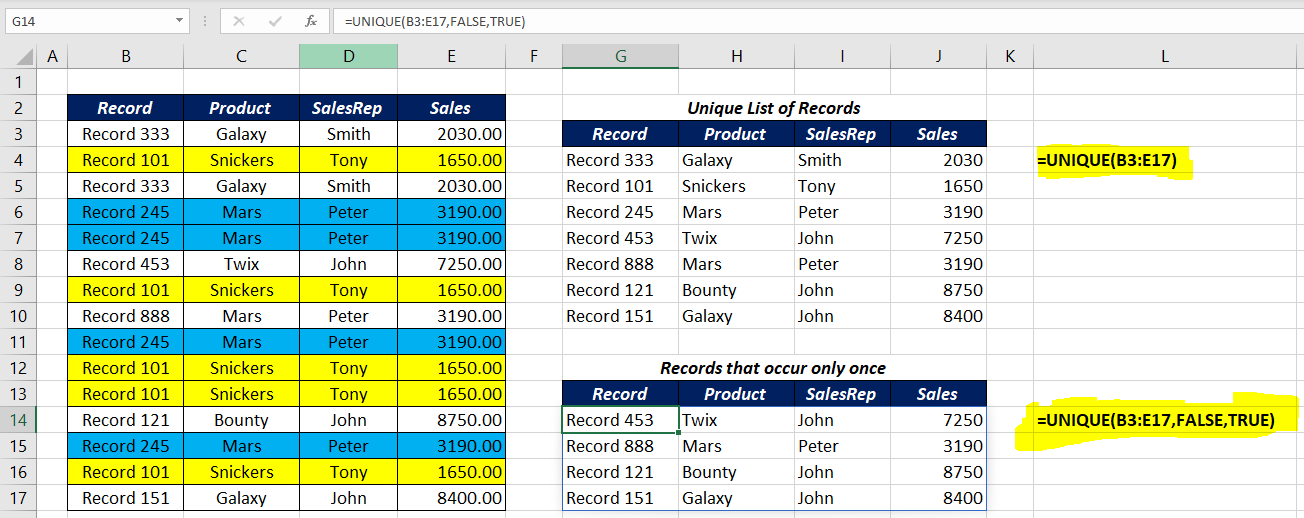# UNIQUE FunctionThe Excel UNIQUE function is a DYNAMIC ARRAY FUNCTION that returns a list of unique values (values that occur only once) from an array or a data range. Values can be numbers, text, dates, etc. The UNIQUE function can return values from single or multiple rows/columns.

### Function Type

Lookup and reference

### Purpose

Extract unique values from a list or range

### Return value

An array of unique values

### Syntax

`=UNIQUE (array, [by_col], [occurs_once])`

### Arguments

array – Range or Array from which to extract unique values.

by_col – [optional], By row = FALSE (default); by column = TRUE.

occurs_once – [optional] FALSE= all unique values (default), TRUE = values that occur once

### Examples

To create a unique list of values in the range B3:B13, use the following formula

`=UNIQUE(B3:B13)`

Another example with a dataset containing text, number, and special characters

To create a unique list of values in the range F3:F13, use the following formula

`=UNIQUE(F3:F13)`

The UNIQUE function can also be used with horizontal data ranges. While dealing with horizontal data ranges, set the value of the second argument (by_col) to 1 or TRUE.

To create a unique list of values present in the horizontal data range C2:M2, use the following formula

`=UNIQUE(C2:M2,TRUE)`

The third argument in the UNIQUE function occurs_once helps us to find the values that occur only once. Set the value of this argument to 1 or TRUE to return the values that occur only once.

In the below example we have a list of items in the data range B3:B13.

To return the unique list of items,

`=UNIQUE(B3:B13)`

and to return the list of items that occur only once,

`=UNIQUE(B3:B13,0,1)`

Once more example with records spanning into multiple columns

To return the unique list of records in the range B3:E17,

`=UNIQUE(B3:E17)`

and to return the records that appear only once in the same data range,

`=UNIQUE(B3:E17,FALSE,TRUE)`

### Note

The Excel UNIQUE function extracts a list of unique values from a range and the result is a dynamic array of values. This array values will ‘spill’ onto the worksheet into a range that automatically updates when new unique values are added to or removed from the source range. So, if this spill range contains any data, the function will return a #SPILL! error.